﻿ 基于喷水推进理论的泵喷推进性能预报研究
 舰船科学技术2020, Vol. 42Issue (6): 39-43    DOI: 10.3404/j.issn.1672-7649.2020.06.008PDF

1. 中国船舶及海洋工程设计研究院 上海 200011;
2. 喷水推进技术重点实验室，上海 200011

Research on the prediction of propulsionperformance of pumpjet based on the theory of waterjet
DONG Xin-guo1,2, LIU Jian-guo1,2, DAI Yuan-xing1,2, SU Wei1
1. Marine Design and Research Institute of China, Shanghai 200011, China;
2. Science and Technology on Water Jet Propulsion Laboratory, Shanghai 200011, China
Abstract: There are mainly two technical methods for the pumpjet design. One is based on the theory of waterjet propulsion, and the other is based on ducted propeller method. This paper established pumpjet control volume theory and the basic equations of pumpjet propulsion, the similarities and distinction between the performance prediction methed based on pumpjet propulsion and waterjet propulsion is analyzed in this paper. The correction coefficient have been researched in combination with the practical parameters. The feasibility and effectiveness of the method are verified through the specific case analysis. It provides reference for the performance prediction and optimization of pumpjet.
Key words: waterjet     pumpjet     propulsion performance
0 引言

“泵喷”（pump jet）和“喷水推进”（water jet）均是由外文直接翻译过来的，water jet一词大致在1661年出现，pump jet一词出现于20世纪20年代，两者原理是一致的，技术核心是“水泵”与“喷射”，都是用泵这种水力机械把水流向后喷射，利用进出流的动量差产生推力。泵喷与我国20世纪广泛应用的喷水推进组合体类似。学术界关于泵喷与喷水推进关系的争论一直存在，王立祥等研究认为：喷水推进和泵喷推进均属泵类推进，都是通过管道内叶轮和导叶的流场匹配设计在喷口产生轴向射流而获得推进作用；喷水推进的第一设计指标是高效率、首要保证快速性，相应的设计结果是喷水推进器；泵喷推进的第一设计指标是低噪声、首要保证声隐身性，相应的设计结果是泵喷推进器。泵喷与喷水推进作用机理和原理一致，技术体系与范畴相同，只是由于应用平台或者设计要求差异带来称谓的不同[1-2]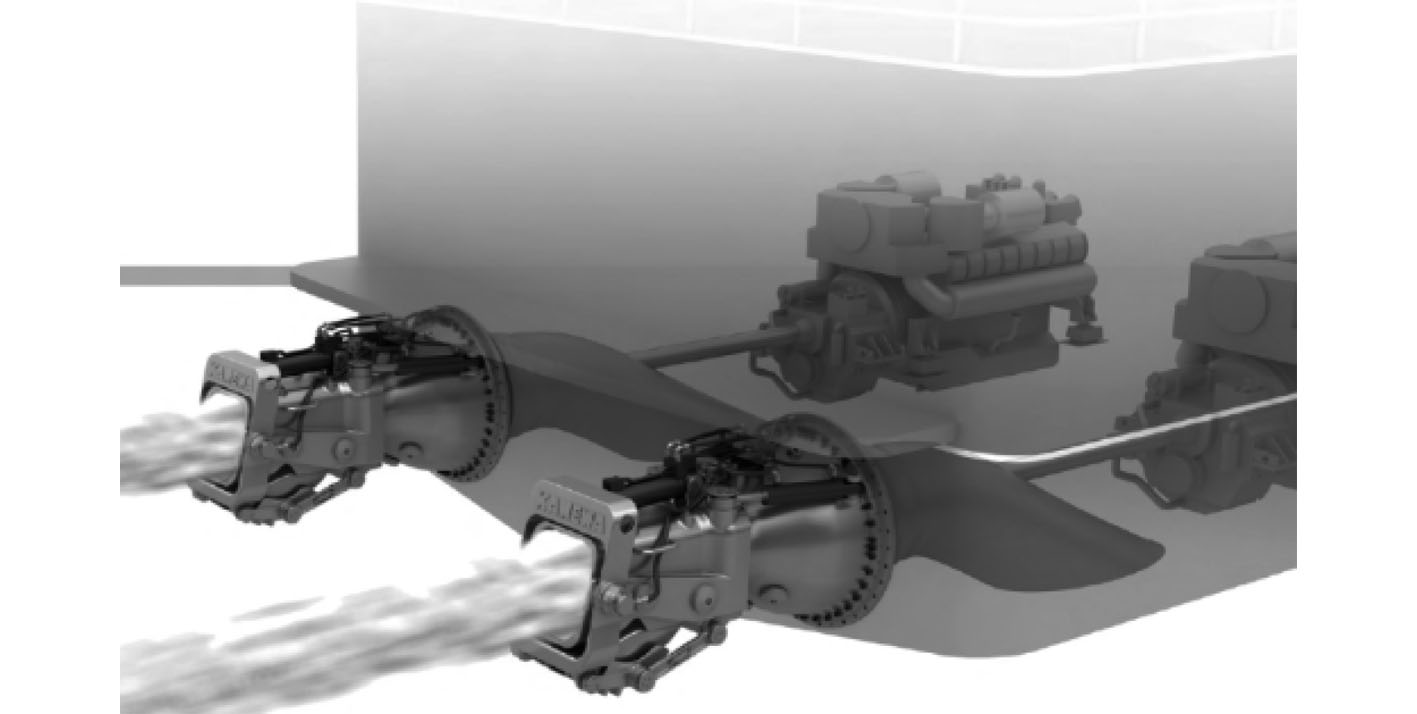图 1 内置式喷水推进装置 Fig. 1 Internal-mounted water jet unit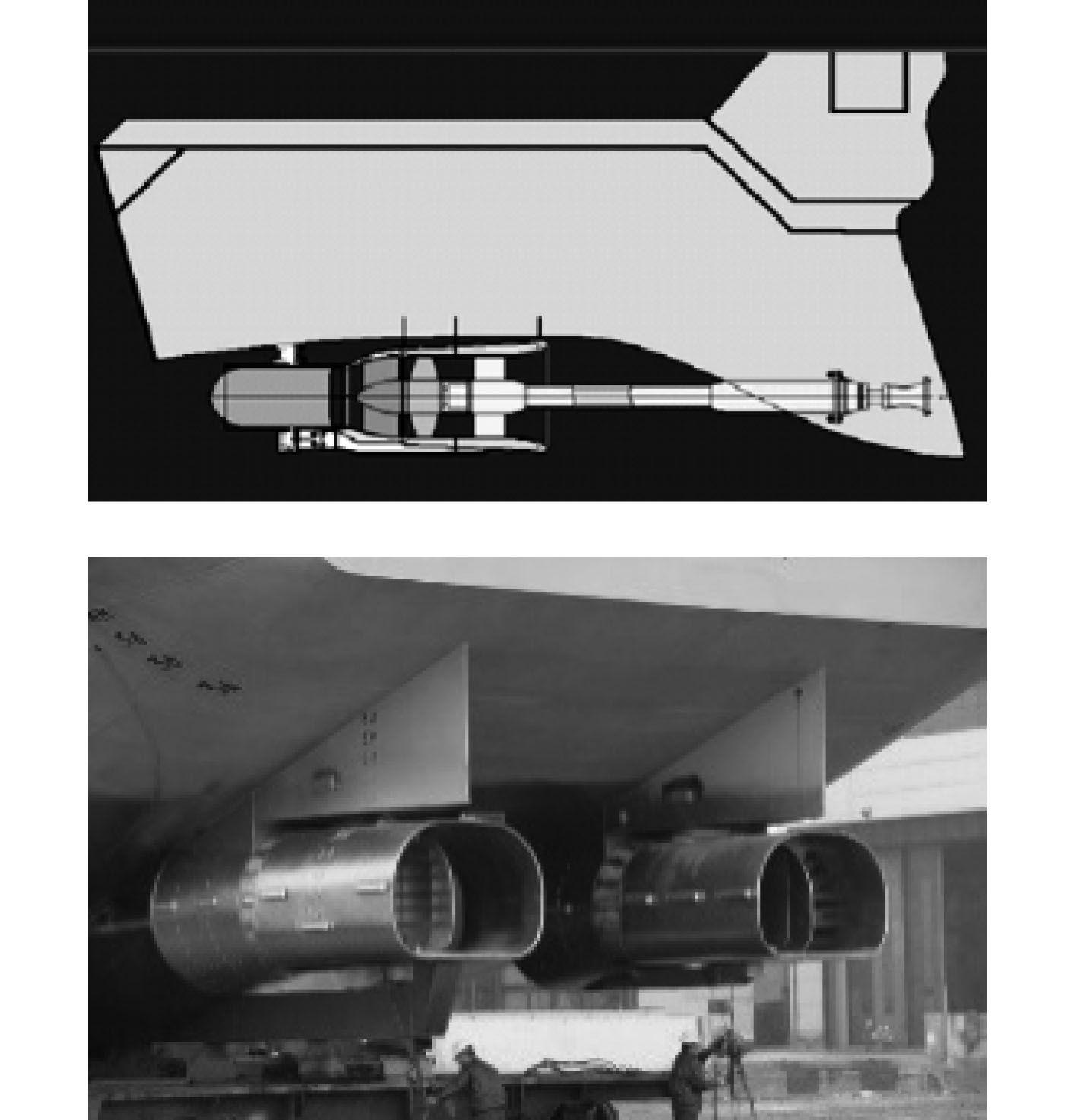图 2 喷水推进组合体（外悬式） Fig. 2 Outer-mounted water jet unit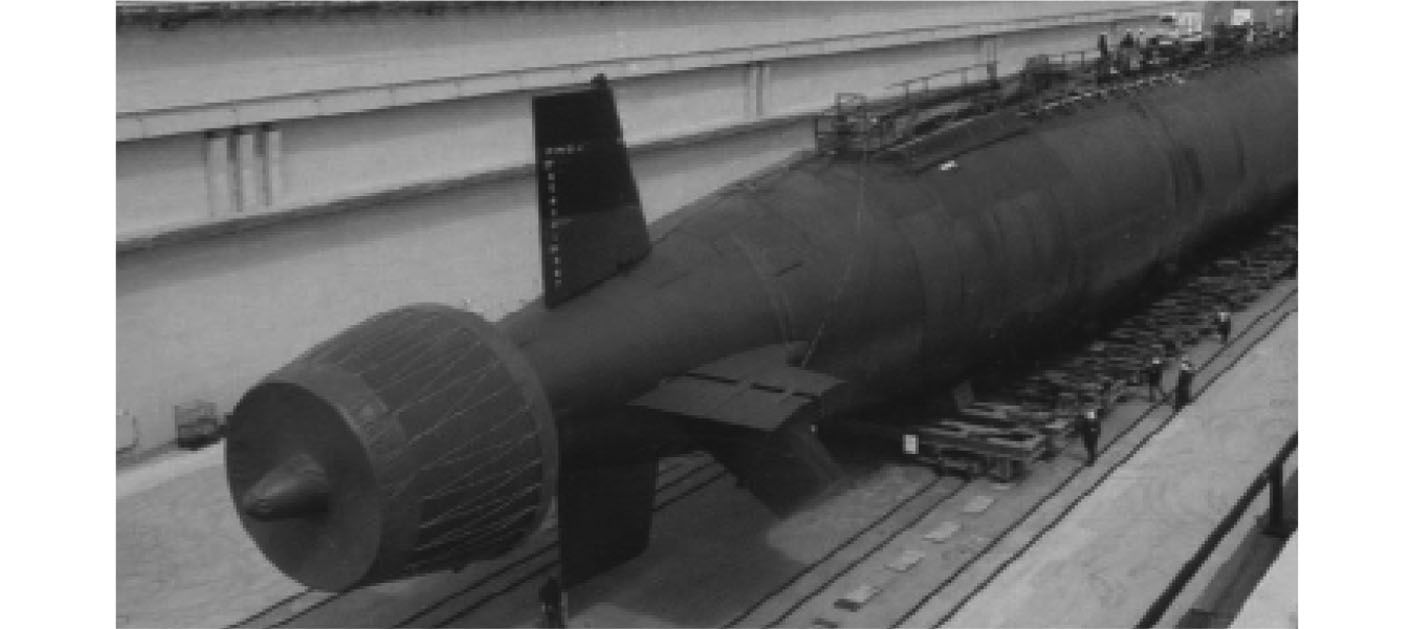图 3 泵喷推进 Fig. 3 Pump jet
1 喷水推进理论及基本方程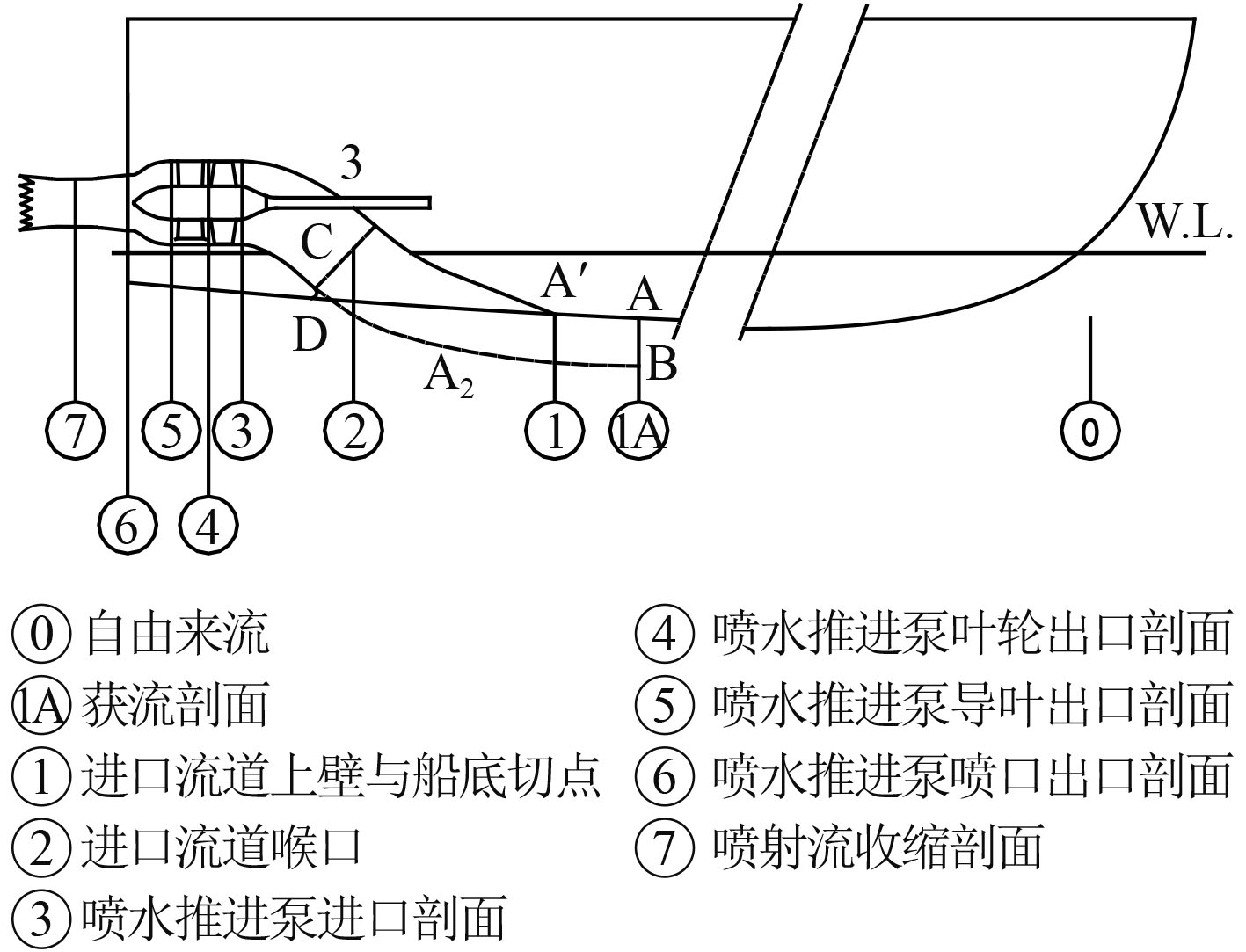图 4 喷水推进系统水动力模型的控制体 Fig. 4 The control volume system of the water jet

1）力平衡方程

 ${T}=\Delta {{M}{x}}_{(7-1{A})}=\rho {Q}{{V}}_{{j}}-\rho {Q}{{V}}_{1{A}}{\text{=}}$
 $\rho {Q}{ ({V}}_{{j}}-\alpha {{V}}_{0}){\text{。}}$ (1)

$\alpha$ 为边界层对动量影响系数，A处获流区面积。

 $\mathrm{\alpha }=\frac{1}{{V}_{0}}\frac{{\int }_{1A}^{}{V}^{2}{\mathrm{d}}A}{{\int }_{1A}^{}V{\mathrm{d}}A}{\text{，}}$ (2)
 $\mathrm{Q}={\int }_{1A}^{}V{\mathrm d}A \text{。}$ (3)

 $R = \left( {1 - t} \right)T \text{。}$ (4)

2）功率平衡方程

 ${\mathrm{N}}_{\mathrm{e}}=\mathrm{\rho }{g}{Q}{H}/{\eta }_{{p}}$ (5)

（3）损失平衡方程

 ${H}=\frac{(1+{k}_{j})}{2g}{{V}_{j}}^{2}+({K}_{1}-\mathrm{\beta })\frac{{{\mathrm{V}}_{0}}^{2}}{2g}+{h}_{c}\text{。}$ (6)

 $\mathrm{\beta }=\frac{1}{{{V}_{0}}^{2}}\frac{{\displaystyle\int }_{1A}^{}{V}^{3}\mathrm dA}{{\displaystyle\int }_{1A}^{}V\mathrm dA}\approx {\alpha }^{2} \text{。}$ (7)
2 泵喷基本方程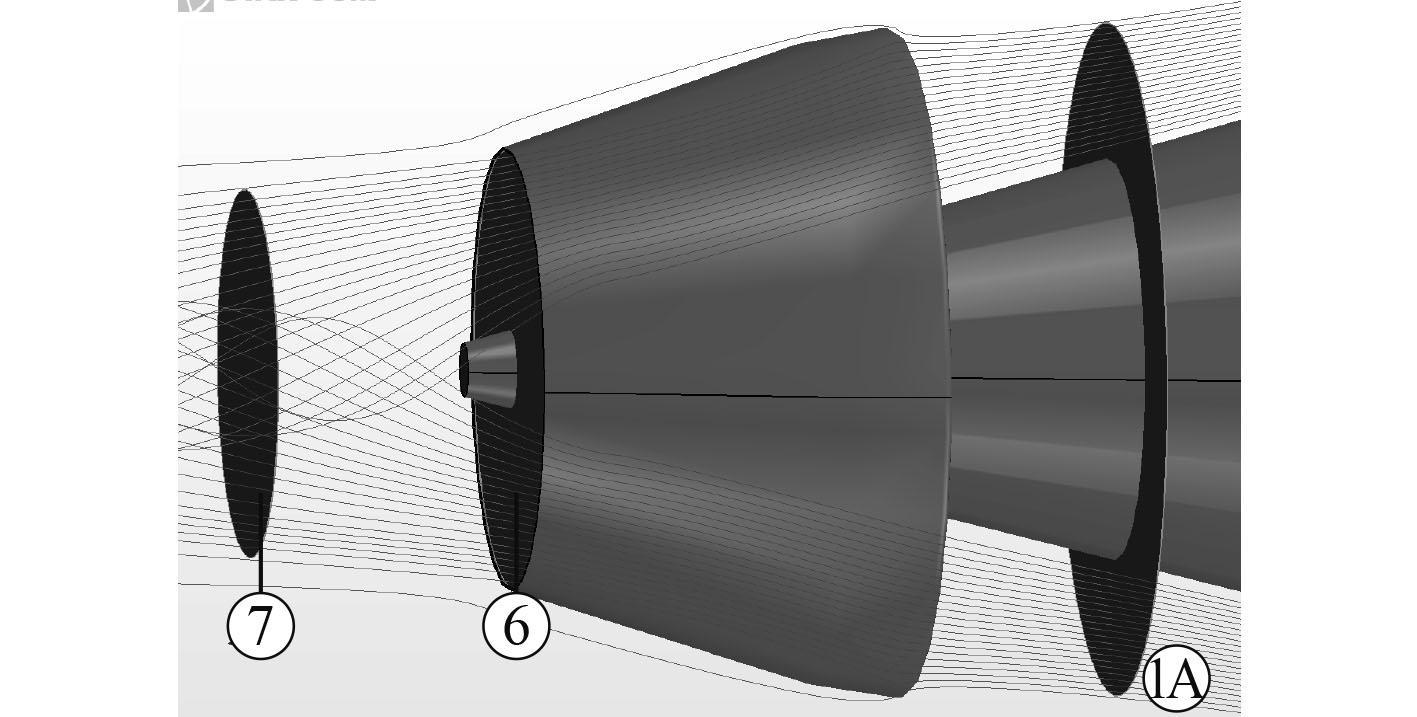图 5 泵喷水动力模型的控制体 Fig. 5 The control volume system of the pumpjet

 ${{T}}'=\Delta {{M}{x}}_{(7'-1\mathrm{A}')}=\rho {Q}{ ({V}}_{{j}}-\alpha {{V}}_{0})\text{。}$ (8)

 ${T}={{T}}'+{T}_{duct}=\rho {Q}{ ({V}}_{{j}}-\alpha {{V}}_{0})+{T}_{\begin{array}{c}duct \\ \end{array}} \text{。}$ (9)

3 喷水推进推进性能预报技术应用于泵喷的差异与修正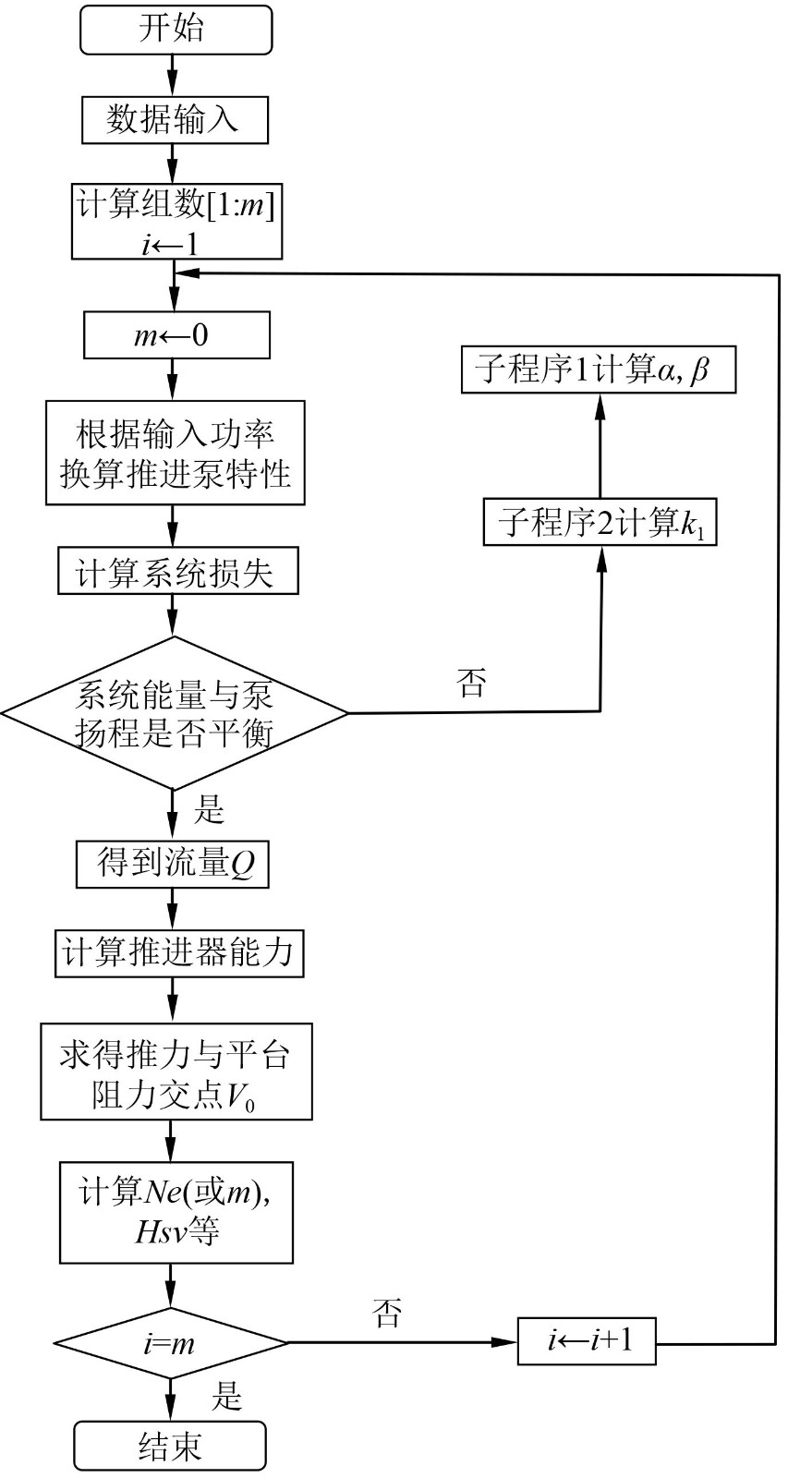图 6 喷水推进性能预报流程 Fig. 6 The procedure of the performance prediction of the waterjet

1）根据推进泵模型试验数据，基于相似准则，确定直径并换算至不同转速n（或功率Ne）下的扬程H与流量Q、效率 ${\eta }_{{p}}$ 与流量Q的关系曲线，即H=fn（或Ne）、Q）、 ${\eta }_{{p}}$ = fn（或Ne）、Q）。

2）按式（5）计算不同航速下的系统损失特性。

3）将不同转速（或功率）下推进泵扬程与不同航速下系统特性（水力损失）的平衡点作为计算依据，按式（3）式、（4）求得不同转速（或功率）下对应的航速、推力和推进泵收到功率（或转速），导入阻力曲线，形成一系列推力与阻力平衡点，对应的HQ ${\eta }_{{p}}$ 即为推进泵的性能。

4）形成喷水推进性能和船舶阻力性能交汇的特性图表

3.1 推进泵模型试验数据应用修正

 ${{H}}_{j}=\mathrm{\lambda }\frac{{L}}{d}\displaystyle\frac{{(\frac{4Q}{\text{π} {d}^{2}})}^{2}}{2g}\text{，}$ (10)

$\mathrm{\lambda }$ 值由下式导出：

 $\frac{1}{\sqrt{\lambda }}=-2{\mathrm{log}}_{10}\left[\frac{2.51}{Re\sqrt{\lambda }}+\frac{1}{74}\right]\text{。}$

3.2 尺度效应修正

 $\frac{{1-{\eta }}_{{h}{s}}}{{1-\mathrm{\eta }}_{{h}{m}}}={\left(\frac{{{D}}_{{m}}}{{{D}}_{{s}}}\right)}^{0.2}\text{，}$ (11)

 $\frac{{{Q_s}}}{{{Q_m}}} = \left( {\frac{{{n_s}}}{{{n_m}}}} \right){\left( {\frac{{{D_s}}}{{{D_m}}}} \right)^3}\text{，}$ (12)
 $\frac{{{H_s}}}{{{H_m}}} = {\left( {\frac{{{n_s}}}{{{n_m}}}} \right)^2}{\left( {\frac{{{D_s}}}{{{D_m}}}} \right)^2}\text{。}$ (13)

 $N{e_s} = \frac{{{\gamma _s}}}{{{\gamma _m}}}{\left( {\frac{{{n_s}}}{{{n_m}}}} \right)^3}{\left( {\frac{{{D_s}}}{{{D_m}}}} \right)^5}N{e_m}\text{，}$ (14)

 $\frac{{1-\mathrm{\eta }}_{\mathrm{h}\mathrm{s}}}{{1-\mathrm{\eta }}_{\mathrm{h}\mathrm{m}}}={\left(\frac{{{D}}_{{m}}}{{{D}}_{{s}}}\right)}^{0.25}{\left(\frac{{H}_{m}}{{H}_{s}}\right)}^{0.1}\text{。}$ (15)

 $\frac{{{Q_s}}}{{Q{}_m}} = {C_Q}\left( {\frac{{{n_s}}}{{{n_m}}}} \right){\left( {\frac{{{D_s}}}{{{D_m}}}} \right)^3}\text{，}$ (16)
 $\frac{{{H_s}}}{{{H_m}}} = {C_H}{\left( {\frac{{{n_s}}}{{{n_m}}}} \right)^2}{\left( {\frac{{{D_s}}}{{{D_m}}}} \right)^2}\text{。}$ (17)

3.3 其他差异性

1）推力

2）空泡

3）边界层影响系数

4 案例分析与验证

1）对应推进泵模型试验数据（见表1图7）；表 1 推进泵模型试验数据（归一化） Tab.1 The model hydrodynamic performance of the waterjet pump（unitary）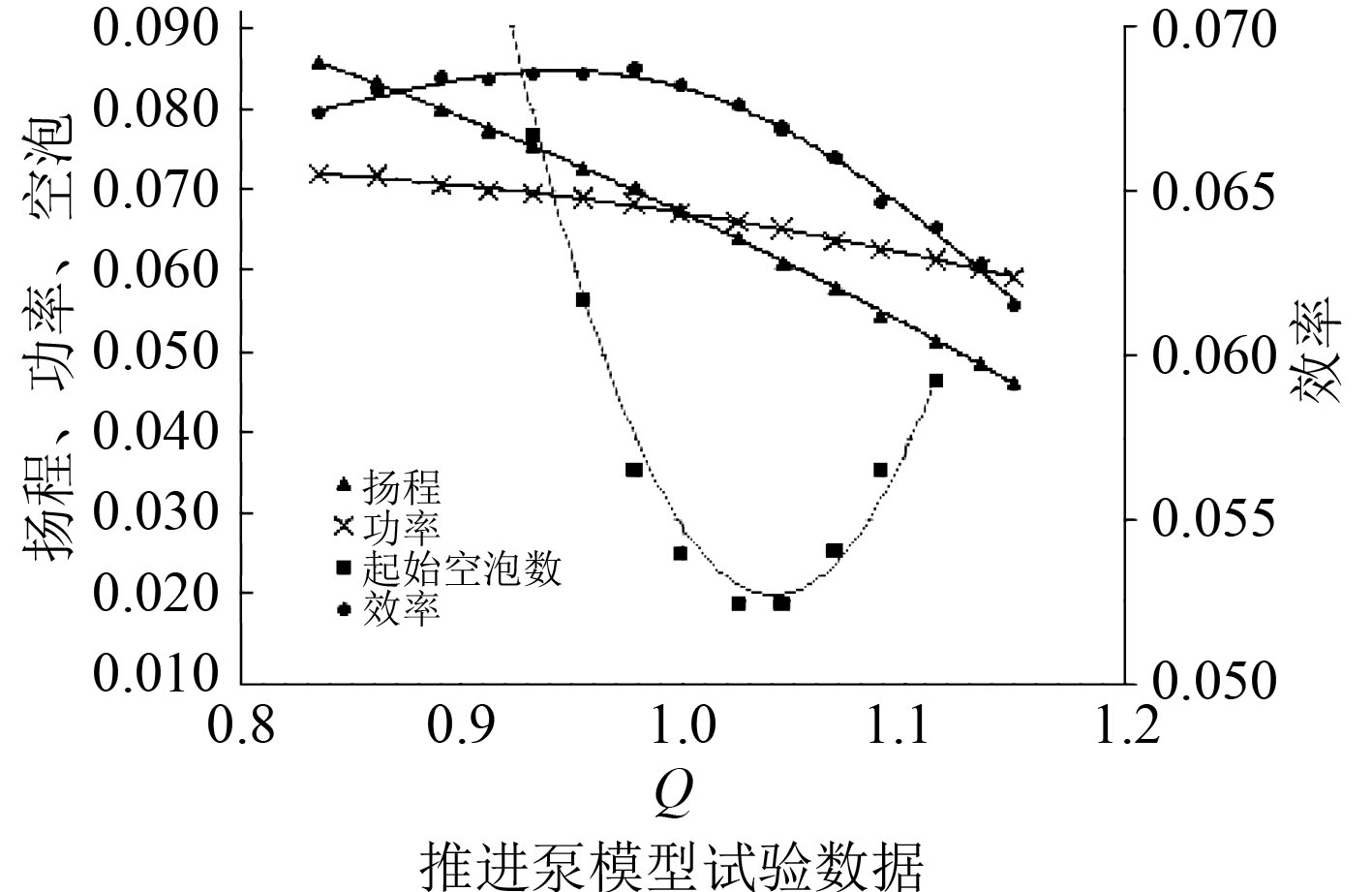图 7 推进泵模型试验曲线 Fig. 7 The model hydrodynamic performance of the waterjet pump

2）艇体阻力曲线；

3）泵喷导管外表面推力曲线；

4）推进性能计算输入参数（见表2）。表 2 泵喷推进性能计算输入参数表 Tab.2 The input forthe prediction of propulsion performance of pumpjet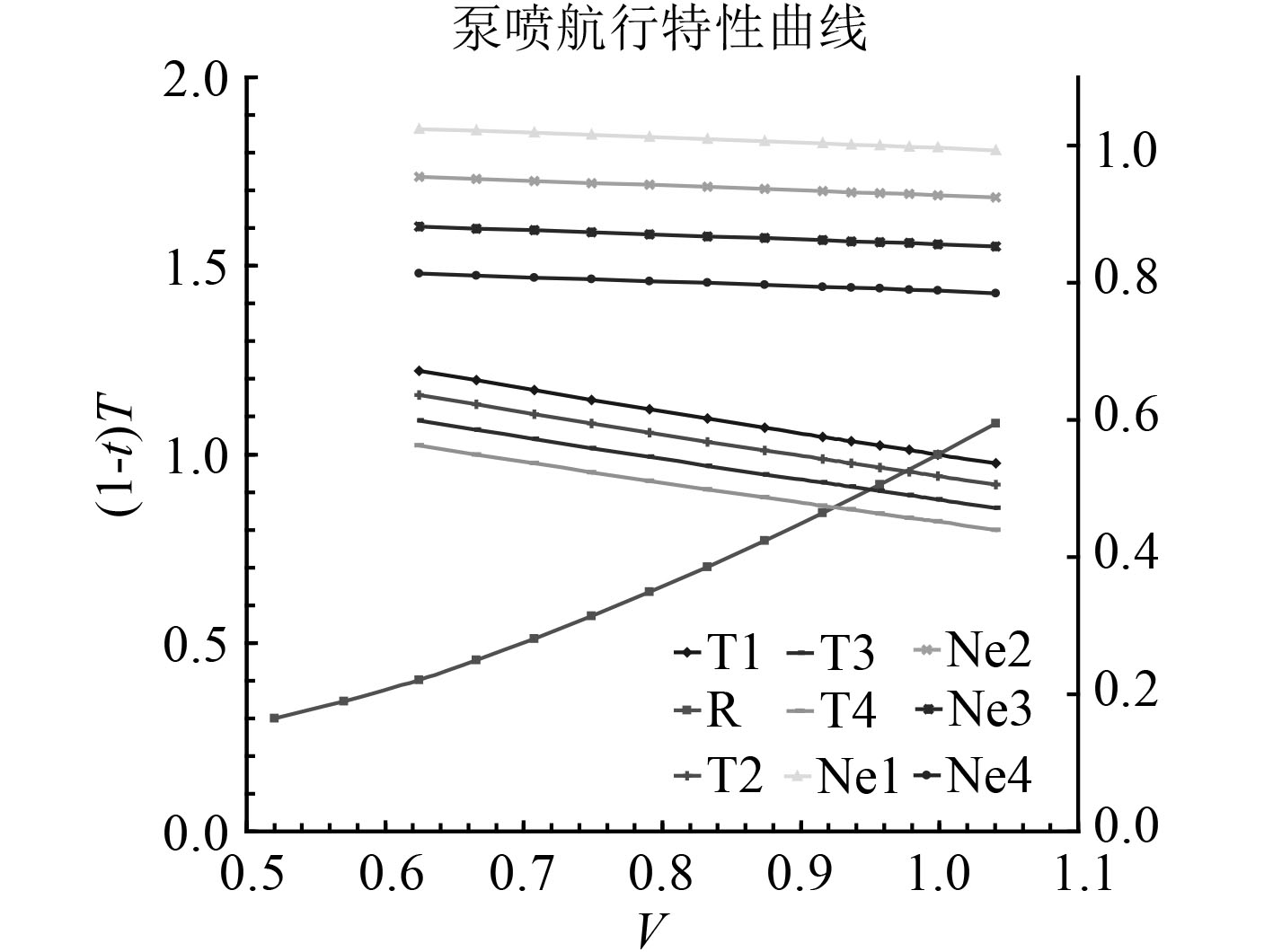图 8 泵喷推进特性曲线（归一化） Fig. 8 The propulsion performance of the pumpjet表 3 推进性能预报与自航试验结果偏差表 Tab.3 The comparison between theperformanceprediction and self-propulsion test
5 结　语

1）本文从喷水推进理论出发，基于动量通量方法，建立了泵喷研究控制体和基本方程；与喷水推进相比，泵喷推力方程增加了导管外表面受力项。

2）泵喷属于高比转速泵范畴，模型泵试验数据应用时需采用测压段管路损失修正扬程和效率曲线；采用Mody3公式换算水力效率、引入流量和扬程修正系数，解决从模型放大至实型时的尺度效应问题。

3）以模型泵试验数据为基础，完成了某泵喷推进性能预报，与泵喷自航试验和艇后空泡试验结果比对显示：匹配转速偏差−0.1%，最高航速偏差−1.6%，空泡临界航速偏差−1.2%，验证了基于喷水推进理论的泵喷推进性能预报方法的可行性和有效性，对泵喷性能预报和设计方法拓展和具有重要意义。

  王立祥、蔡佑林. 喷水推进及推进泵设计理论和技术[M]. 上海: 上海交通大学出版, 2018: 1-12, 73-90.  王永生. 喷水推进和泵喷推进的概念: 共性、特性及区别[J]. 中国舰船研究, 2019, 5: 1-10.  王天奎, 唐登海. 泵喷推进器—低噪声的核潜艇推进方式[J]. 现代军事, 2006(7): 52-54.  魏以迈. 潜艇低噪声推进器技术研究与进展[C]//第十届船舶水下噪声学术讨论会论文集. 2005: 15-18.  KRUPPA, C. (Chairman). Report of the specialist committee on waterjets[C]//21st ITTC, Bergen and Trondheim, 1996.  HOYT III, J. G. (Chairman). Report of the specialist committee on waterjet[C]//22nd ITTC, Seoul/Shanghai, 1999.  VAN TERWISGA, T. J. C. (Chairman). Report of the specialist committee on validation of waterjet test procedures[C]//23rd-24 rd ITTC, Venice, Volume II,2002-2005.  金平仲. 船舶喷水推进[M]. 北京: 国防工业出版社, 1986: 67-104.  陈松山, 严登丰, 葛强. 泵及泵装置效率换算方法研究[J]. 农业机械学报, 2006, 37: 52-55, 59. DOI:10.3969/j.issn.1000-1298.2006.08.013  戴原星, 王立祥. 基于CFD的前置导叶轴流泵通用特性曲线预报较[J]. 船舶, 2013, 10: 1-5. DOI:10.3969/j.issn.1001-9855.2013.04.001  孙存楼, 王永生. 喷水推进推力预报的两种不同方法比较[J]. 船舶力学, 2010, 11: 1208-1212. DOI:10.3969/j.issn.1007-7294.2010.11.003